<![CDATA[Journal of Plasma Physics]]>https://www.cambridge.org/core/journals/journal-of-plasma-physics/latest-issueJournal of Plasma Physics0022377814697807Cambridge University Press<![CDATA[Multi-scale dynamics of magnetic flux tubes and inverse magnetic energy transfer]]>

We report on an analytical and numerical study of the dynamics of a three-dimensional array of identical magnetic flux tubes in the reduced-magnetohydrodynamic description of the plasma. We propose that the long-time evolution of this system is dictated by flux-tube mergers, and that such mergers are dynamically constrained by the conservation of the pertinent (ideal) invariants, viz. the magnetic potential and axial fluxes of each tube. We also propose that in the direction perpendicular to the merging plane, flux tubes evolve in a critically balanced fashion. These notions allow us to construct an analytical model for how quantities such as the magnetic energy and the energy-containing scale evolve as functions of time. Of particular importance is the conclusion that, like its two-dimensional counterpart, this system exhibits an inverse transfer of magnetic energy that terminates only at the system scale. We perform direct numerical simulations that confirm these predictions and reveal other interesting aspects of the evolution of the system. We find, for example, that the early time evolution is characterized by a sharp decay of the initial magnetic energy, which we attribute to the ubiquitous formation of current sheets. We also show that a quantitatively similar inverse transfer of magnetic energy is observed when the initial condition is a random, small-scale magnetic seed field.

]]>
https://dx.doi.org/10.1017/S0022377820000641?rft_dat=source%3Ddrssdoi:10.1017/S0022377820000641Multi-scale dynamics of magnetic flux tubes and inverse magnetic energy transfer53586040110.1017/S0022377820000641https://dx.doi.org/10.1017/S0022377820000641?rft_dat=source%3Ddrss2020-07-08Journal of Plasma Physics2020-07-08Zhou, MuniLoureiro, Nuno F.Uzdensky, Dmitri A.
<![CDATA[Progress on the small modular stellarator SCR-1: new diagnostics and heating scenarios]]>

This work presents updates in the diagnostics systems, magnetohydrodynamics (MHD) calculations and simulations of microwave heating scenarios of the small modular Stellarator of Costa Rica 1 (SCR-1). Similarly, the design of a flexible bolometer and magnetic diagnostics (a set of Mirnov coils, Rogowski coils and two diamagnetic loops) are introduced. Furthermore, new MHD equilibrium calculations for the plasma of the SCR-1 device were performed using the VMEC code including the poloidal cross-section of the magnetic flux surfaces at different toroidal positions, profiles of the rotational transform, magnetic well, magnetic shear and total magnetic field norm. Charged particle orbits in vacuum magnetic field were computed by the magnetic field solver BS-SOLCTRA (Vargas et al. In 27th IAEA Fusion Energy Conference (FEC 2018), 2018. IAEA). A visualization framework was implemented using Paraview (Solano-Piedra et al. In 23rd IAEA Technical Meeting on the Research Using Small Fusion Devices (23rd TM RUSFD), 2017) and compared with magnetic mapping results (Coto-Vílchez et al. In 16th Latin American Workshop on Plasma Physics (LAWPP), 2017, pp. 43–46). Additionally, simulations of microwave heating scenarios were performed by the IPF-FDMC full-wave code. These simulations calculate the conversion of the ordinary waves to extraordinary waves and allow us to identify the location where the conversion takes place. Finally, the microwave heating scenarios for the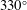toroidal position are presented. The microwave heating scenarios showed that the O–X–B mode conversion is around 12–14 %. It was possible to identify the spatial zone where the conversion takes place (upper hybrid frequency).

]]>
https://dx.doi.org/10.1017/S0022377820000677?rft_dat=source%3Ddrssdoi:10.1017/S0022377820000677Progress on the small modular stellarator SCR-1: new diagnostics and heating scenarios81586040110.1017/S0022377820000677https://dx.doi.org/10.1017/S0022377820000677?rft_dat=source%3Ddrss2020-07-08Journal of Plasma Physics2020-07-08Coto-Vílchez, F.Vargas, V. I.Solano-Piedra, R.Rojas-Quesada, M. A.Araya-Solano, L. A.Ramírez, A. A.Hernández-Cisneros, M.Pérez-Hidalgo, J. E.Köhn-Seemann, A.Cerdas, F.Vílchez-Coto, F.Jiménez, D.Campos-Duarte, L.Meneses, E.González-Vega, M.Arias, S.
<![CDATA[Semi-analytic model for the electromagnetic field of a current-driven antenna in a cold, magnetized plasma]]>

In this semi-analytic study we develop a mathematical model for determining the electromagnetic field due to a current-driven antenna immersed in a cold, magnetized plasma, valid for frequencies below the electron plasma frequency. At each point in the plasma, it is shown that the vacuum electric field of the antenna couples to the plasma conductivity tensor and acts as an infinitesimal source term to drive plasma currents – the total field is then found from the aggregate sum of these point sources, expressed as an integral across the vacuum field. A general solution is provided for both azimuthally symmetric cylindrical coordinates as well as a fully generalized Cartesian solution. As an example of how this general solution may be applied, we solve for the field due to an electric dipole antenna of length, aligned along the background field, at frequencies below the ion cyclotron frequency. It is found that the near field decays exponentially with increasing, whereas the far field exhibits wave-like behaviour. The radiation zone exhibits propagation cones emanating from either end of the dipole, with a propagation angle that is consistent with past analytic studies of inertial Alfvén waves. The mathematical model presented here may be advantageous over other numerical methods, as it allows the user to solve parts of the problems analytically, thereby cutting down significantly on computation time, as well as offering physical insight into the system that may not be evident with other numerical solvers.

]]>
https://dx.doi.org/10.1017/S0022377820000446?rft_dat=source%3Ddrssdoi:10.1017/S0022377820000446Semi-analytic model for the electromagnetic field of a current-driven antenna in a cold, magnetized plasma83586040110.1017/S0022377820000446https://dx.doi.org/10.1017/S0022377820000446?rft_dat=source%3Ddrss2020-07-24Journal of Plasma Physics2020-07-24Robertson, J.
<![CDATA[Solving gyrokinetic systems with higher-order time dependence]]>

We discuss theoretical and numerical aspects of gyrokinetics as a Lagrangian field theory when the field perturbation is introduced into the symplectic part. A consequence is that the field equations and particle equations of motion in general depend on the time derivatives of the field. The most well-known example is when the parallel vector potential is introduced as a perturbation, where a time derivative of the field arises only in the equations of motion, so an explicit equation for the fields may still be written. We will consider the conceptually more problematic case where the time-dependent fields appear in both the field equations and equations of motion, but where the additional term in the field equations is formally small. The conceptual issues were described by Burby (J. Plasma Phys., vol. 82 (3), 2016, 905820304): these terms lead to apparent additional degrees of freedom to the problem, so that the electric field now requires an initial condition, which is not required in low-frequency (Darwin) Vlasov–Maxwell equations. Also, the small terms in the Euler–Lagrange equations are a singular perturbation, and these two issues are interlinked. For well-behaved problems the apparent additional degrees of freedom are spurious, and the physically relevant solution may be directly identified. Because we needed to assume that the system is well behaved for small perturbations when deriving gyrokinetic theory, we must continue to assume that when solving it, and the physical solutions are thus the regular ones. The spurious nature of the singular degrees of freedom may also be seen by changing coordinate systems so the varying field appears only in the Hamiltonian. We then describe how methods appropriate for singular perturbation theory may be used to solve these asymptotic equations numerically. We then describe a proof-of-principle implementation of these methods for an electrostatic strong-flow gyrokinetic system; two basic test cases are presented to illustrate code functionality.

]]>
https://dx.doi.org/10.1017/S0022377820000653?rft_dat=source%3Ddrssdoi:10.1017/S0022377820000653Solving gyrokinetic systems with higher-order time dependence90586040110.1017/S0022377820000653https://dx.doi.org/10.1017/S0022377820000653?rft_dat=source%3Ddrss2020-07-16Journal of Plasma Physics2020-07-16Sharma, A. Y.McMillan, B. F.
<![CDATA[Conservative discontinuous Galerkin schemes for nonlinear Dougherty–Fokker–Planck collision operators]]>

We present a novel discontinuous Galerkin algorithm for the solution of a class of Fokker–Planck collision operators. These operators arise in many fields of physics, and our particular application is for kinetic plasma simulations. In particular, we focus on an operator often known as the ‘Lenard–Bernstein’ or ‘Dougherty’ operator. Several novel algorithmic innovations, based on the concept of weak equality, are reported. These weak equalities are used to define weak operators that compute primitive moments, and are also used to determine a reconstruction procedure that allows an efficient and accurate discretization of the diffusion term. We show that when two integrations by parts are used to construct the discrete weak form, and finite velocity-space extents are accounted for, a scheme that conserves density, momentum and energy exactly is obtained. One novel feature is that the requirements of momentum and energy conservation lead to unique formulas to compute primitive moments. Careful definition of discretized moments also ensure that energy is conserved in the piecewise linear case, even though the kinetic-energy term,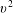is not included in the basis set used in the discretization. A series of benchmark problems is presented and shows that the scheme conserves momentum and energy to machine precision. Empirical evidence also indicates that entropy is a non-decreasing function. The collision terms are combined with the Vlasov equation to study collisional Landau damping and plasma heating via magnetic pumping.

]]>
https://dx.doi.org/10.1017/S0022377820000586?rft_dat=source%3Ddrssdoi:10.1017/S0022377820000586Conservative discontinuous Galerkin schemes for nonlinear Dougherty–Fokker–Planck collision operators90586040310.1017/S0022377820000586https://dx.doi.org/10.1017/S0022377820000586?rft_dat=source%3Ddrss2020-07-17Journal of Plasma Physics2020-07-17Hakim, AmmarFrancisquez, ManaureJuno, JamesHammett, Gregory W.
<![CDATA[Diagnosing collisionless energy transfer using field–particle correlations: Alfvén-ion cyclotron turbulence]]>

We apply field–particle correlations – a technique that tracks the time-averaged velocity-space structure of the energy density transfer rate between electromagnetic fields and plasma particles – to data drawn from a hybrid Vlasov–Maxwell simulation of Alfvén-ion cyclotron turbulence. Energy transfer in this system is expected to include both Landau and cyclotron wave–particle resonances, unlike previous systems to which the field–particle correlation technique has been applied. In this simulation, the energy transfer rate mediated by the parallel electric field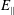comprises approximately 60 % of the total rate, with the remainder mediated by the perpendicular electric field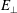. The parallel electric field resonantly couples to protons, with the canonical bipolar velocity-space signature of Landau damping identified at many points throughout the simulation. The energy transfer mediated bypreferentially couples to particles with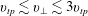, where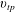is the proton thermal speed, in agreement with the expected formation of a cyclotron diffusion plateau. Our results demonstrate clearly that the field–particle correlation technique can distinguish distinct channels of energy transfer using single-point measurements, even at points in which multiple channels act simultaneously, and can be used to determine quantitatively the rates of particle energization in each channel.

]]>
https://dx.doi.org/10.1017/S0022377820000689?rft_dat=source%3Ddrssdoi:10.1017/S0022377820000689Diagnosing collisionless energy transfer using field–particle correlations: Alfvén-ion cyclotron turbulence90586040210.1017/S0022377820000689https://dx.doi.org/10.1017/S0022377820000689?rft_dat=source%3Ddrss2020-07-24Journal of Plasma Physics2020-07-24Klein, Kristopher G.Howes, Gregory G.TenBarge, Jason M.Valentini, Francesco